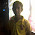# Different Types Of Errors In C

Errors are mistake that we programmer often commit. Errors also called as bugs causes the program to either run unexpectedly ( shows unexpected result ) or prevent the execution of a program. As programmer we are prone to make mistakes. So we should keep in mind the following different types of errors in c which we might commit :

## SYNTAX ERROR IN C

• Syntax Errors are basically compiler errors or compile-time errors which occurs when we do not follow the grammar of the programming language.
• It is detected when you compile the program by the compiler.

Syntax Errors occurs :
• Due to missing semicolon ( ' ; ' ). It is used as an terminating statement. So when you forget to place a semicolon or use any other alternative then it causes syntax error.
```   #include<stdio.h>
int main()
{
int a=10 // Syntax error as semicolon is missing
int b=100 : // Syntax error as using ':' instead of ';'
return 0;
}
```
• It occurs when we use a variable which is not declared in program.
• ```  #include<stdio.h>
int main()
{
printf("Value of a : %d",a); // error as 'a' is not declared anywhere in program
return 0;
}
```
• Error Occurs due to missing and unmatched parenthesis.
```  // Syntax Error as ')' parenthesis is missing
void sum(

// Syntax Error as '}' parenthesis is missing
void product()
{ statement

// Syntax Error due to unmatched parenthesis
void a{}
```

## SEMANTIC ERROR IN C

• Semantic errors occurs when the statement written in the program are not meaningful to the compiler.
• Semantic errors indicate an improper use of Java statements.
Example : int a+b=c; // Semantic Error
Correct one : int c=a+b;

int a=+b; // Semantic Error
Correct one : int a+=b; // Shorthand notation

## LOGICAL ERROR IN C

• Logical errors are errors that shows unexpected results.
• It occurs when you write a program that works, but does not do what you intend it to do.
Example : Suppose you want to find the factorial of a number but instead of multiplying number you end up adding it.
```#include<stdio.h>
int main()
{
int i;
int factorial=1;

for(i=1;i<=n;i++)
factorial=factorial+i; // Logical Error. Correct statement : factorial=factorial*i;

return 0;
}
```

Though the program would work fine and would not show any syntax or run time error. But the output which you were expecting will not be shown.

• Linker Errors are errors that occurs when we do not include header files for the predefined functions used in a program and when we  misspell a standard c function.
```#include<stdio.h>
int Main() // Linker error as 'main' is misspelled as 'Main'
{
printf("Hello : ");
return 0;
}
```

## RUNTIME ERROR IN C

• Run-time errors are errors that occurs during the execution of the program.
Example :
• When we divide a number by zero.
• When input data is not in the correct format.
```//Runtime error program in c
#include<stdio.h>
int main()
{
int a=10,b=0,result;
int number;

result=a/b; // Runtime error
scanf("%d",&number); // Error occurs when you input some other character instead of 'numbers'

return 0;
}
```

#### Related Posts

Previous
Next Post »